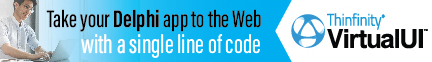DelphiBasicsNaNConstant Not a real number Math unit
 const NaN = 0.0 / 0.0;
Description
The NaN constant provides a standard undetrmined number value for assigning to floating point variables.

It allows the floating point number to be treated as unassigned.
Related commands
 Infinity Floating point value of infinite size IsInfinite Checks whether a floating point number is infinite IsNaN Checks to see if a floating point number holds a real number
Author linksDownload this web site as a Windows program.

 Example code : Assign NAN to a number and then test using IsNaN var   float : Double; begin  // Set the number to an invalid number  float := NaN;     // Equivalent to 0.0/0.0   // Although an invalid number, we can still display it   ShowMessage('float = '+FloatToStr(float));   // And we can test to see if it is a valid number   if IsNaN(float)   then ShowMessage('float is not a number')   else ShowMessage('float = '+FloatToStr(float)); end; Show full unit code float = NAN   float is not a number
 Delphi Programming © Neil Moffatt 2002 - 2020. All rights reserved.  |  Home Page